GFG App
Open AppBrowser
Continue

# Banker’s Algorithm in Operating System

The banker’s algorithm is a resource allocation and deadlock avoidance algorithm that tests for safety by simulating the allocation for the predetermined maximum possible amounts of all resources, then makes an “s-state” check to test for possible activities, before deciding whether allocation should be allowed to continue.

## Why Banker’s algorithm is named so?

Banker’s algorithm is named so because it is used in the banking system to check whether a loan can be sanctioned to a person or not. Suppose there are n number of account holders in a bank and the total sum of their money is S. If a person applies for a loan then the bank first subtracts the loan amount from the total money that the bank has and if the remaining amount is greater than S then only the loan is sanctioned. It is done because if all the account holders come to withdraw their money then the bank can easily do it.

In other words, the bank would never allocate its money in such a way that it can no longer satisfy the needs of all its customers. The bank would try to be in a safe state always.

The following Data structures are used to implement the Banker’s Algorithm:
Let ‘n’ be the number of processes in the system and ‘m’ be the number of resource types.
Available :

• It is a 1-d array of size ‘m’ indicating the number of available resources of each type.
• Available[ j ] = k means there are ‘k’ instances of resource type Rj

Max :

• It is a 2-d array of size ‘n*m’ that defines the maximum demand of each process in a system.
• Max[ i, j ] = k means process Pi may request at most ‘k’ instances of resource type Rj.

Allocation :

• It is a 2-d array of size ‘n*m’ that defines the number of resources of each type currently allocated to each process.
• Allocation[ i, j ] = k means process Pi is currently allocated ‘k’ instances of resource type Rj

Need :

• It is a 2-d array of size ‘n*m’ that indicates the remaining resource need of each process.
• Need [ i,   j ] = k means process Pi currently needs ‘k’ instances of resource type Rj
• Need [ i,   j ] = Max [ i,   j ] – Allocation [ i,   j ]

Allocation specifies the resources currently allocated to process Pi and Needi specifies the additional resources that process Pi may still request to complete its task.
Banker’s algorithm consists of a Safety algorithm and a Resource request algorithm.

## Safety Algorithm

The algorithm for finding out whether or not a system is in a safe state can be described as follows:

1) Let Work and Finish be vectors of length ‘m’ and ‘n’ respectively.
Initialize: Work = Available
Finish[i] = false; for i=1, 2, 3, 4….n
2) Find an i such that both
a) Finish[i] = false
b) Needi <= Work
if no such i exists goto step (4)
3) Work = Work + Allocation[i]
Finish[i] = true
goto step (2)
4) if Finish [i] = true for all i
then the system is in a safe state

Resource-Request Algorithm
Let Requesti be the request array for process Pi. Requesti [j] = k means process Pi wants k instances of resource type Rj. When a request for resources is made by process Pi, the following actions are taken:

1) If Requesti <= Needi
Goto step (2) ; otherwise, raise an error condition, since the process has exceeded its maximum claim.
2) If Requesti <= Available
Goto step (3); otherwise, Pi must wait, since the resources are not available.
3) Have the system pretend to have allocated the requested resources to process Pi by modifying the state as
follows:
Available = Available – Requesti
Allocationi = Allocationi + Requesti
Needi = Needi– Requesti

Example:
Considering a system with five processes P0 through P4 and three resources of type A, B, C. Resource type A has 10 instances, B has 5 instances and type C has 7 instances. Suppose at time t0 following snapshot of the system has been taken: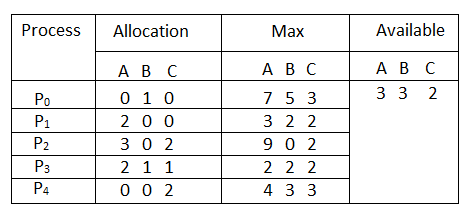Question1. What will be the content of the Need matrix?
Need [i, j] = Max [i, j] – Allocation [i, j]
So, the content of Need Matrix is: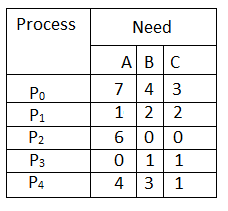Question2.  Is the system in a safe state? If Yes, then what is the safe sequence?
Applying the Safety algorithm on the given system,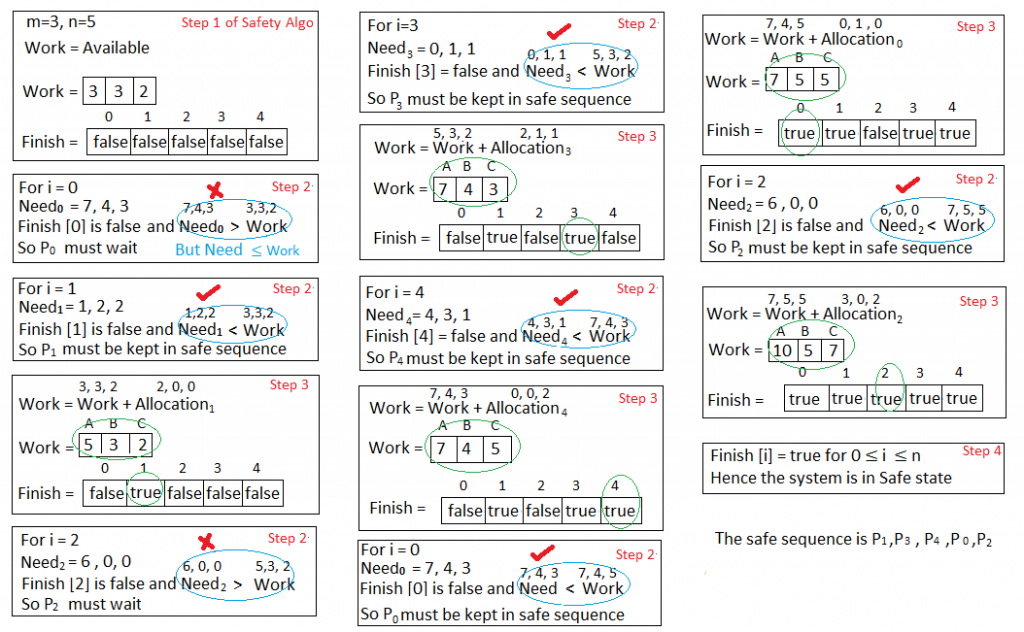Question3. What will happen if process Prequests one additional instance of resource type A and two instances of resource type C?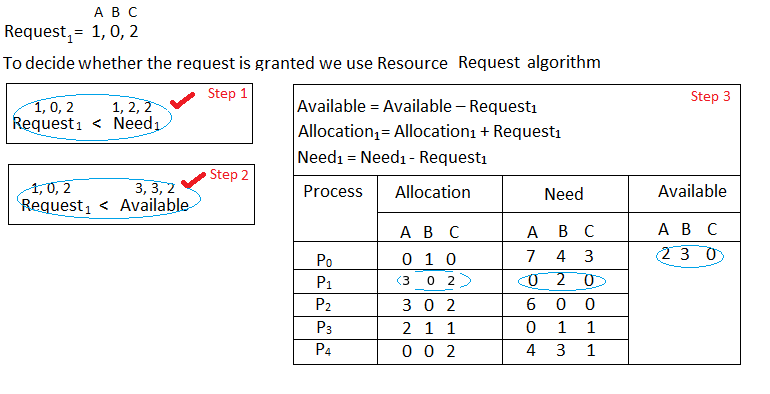We must determine whether this new system state is safe. To do so, we again execute Safety algorithm on the above data structures.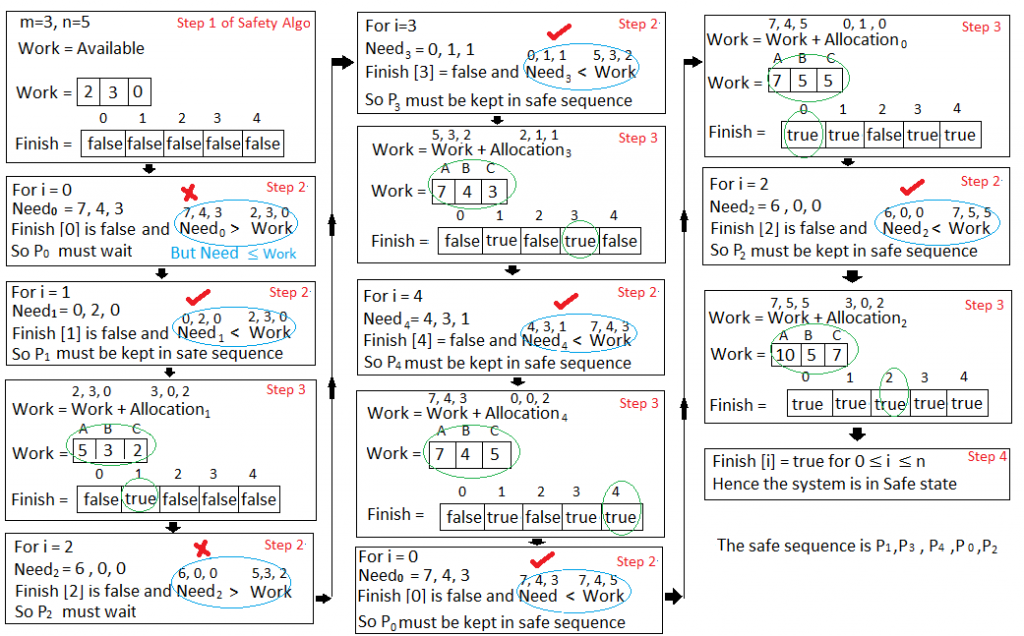Hence the new system state is safe, so we can immediately grant the request for process  P1 .
Code for Banker’s Algorithm

## C

 `// Banker's Algorithm` `#include ` `int` `main()` `{` `    ``// P0, P1, P2, P3, P4 are the Process names here`   `    ``int` `n, m, i, j, k;` `    ``n = 5; ``// Number of processes` `    ``m = 3; ``// Number of resources` `    ``int` `alloc = { { 0, 1, 0 }, ``// P0    // Allocation Matrix` `                        ``{ 2, 0, 0 }, ``// P1` `                        ``{ 3, 0, 2 }, ``// P2` `                        ``{ 2, 1, 1 }, ``// P3` `                        ``{ 0, 0, 2 } }; ``// P4`   `    ``int` `max = { { 7, 5, 3 }, ``// P0    // MAX Matrix` `                      ``{ 3, 2, 2 }, ``// P1` `                      ``{ 9, 0, 2 }, ``// P2` `                      ``{ 2, 2, 2 }, ``// P3` `                      ``{ 4, 3, 3 } }; ``// P4`   `    ``int` `avail = { 3, 3, 2 }; ``// Available Resources`   `    ``int` `f[n], ans[n], ind = 0;` `    ``for` `(k = 0; k < n; k++) {` `        ``f[k] = 0;` `    ``}` `    ``int` `need[n][m];` `    ``for` `(i = 0; i < n; i++) {` `        ``for` `(j = 0; j < m; j++)` `            ``need[i][j] = max[i][j] - alloc[i][j];` `    ``}` `    ``int` `y = 0;` `    ``for` `(k = 0; k < 5; k++) {` `        ``for` `(i = 0; i < n; i++) {` `            ``if` `(f[i] == 0) {`   `                ``int` `flag = 0;` `                ``for` `(j = 0; j < m; j++) {` `                    ``if` `(need[i][j] > avail[j]){` `                        ``flag = 1;` `                         ``break``;` `                    ``}` `                ``}`   `                ``if` `(flag == 0) {` `                    ``ans[ind++] = i;` `                    ``for` `(y = 0; y < m; y++)` `                        ``avail[y] += alloc[i][y];` `                    ``f[i] = 1;` `                ``}` `            ``}` `        ``}` `    ``}` `  `  `      ``int` `flag = 1;` `      `  `      ``for``(``int` `i=0;i"``, ans[i]);` `      ``printf``(``" P%d"``, ans[n - 1]);` `    ``}` `    `    `    ``return` `(0);`   `    ``// This code is contributed by Deep Baldha (CandyZack)` `}`

## C++

 `// Banker's Algorithm` `#include ` `using` `namespace` `std;`   `int` `main()` `{` `    ``// P0, P1, P2, P3, P4 are the Process names here`   `  ``int` `n, m, i, j, k;` `  ``n = 5; ``// Number of processes` `  ``m = 3; ``// Number of resources` `  ``int` `alloc = { { 0, 1, 0 }, ``// P0 // Allocation Matrix` `                     ``{ 2, 0, 0 }, ``// P1` `                     ``{ 3, 0, 2 }, ``// P2` `                     ``{ 2, 1, 1 }, ``// P3` `                     ``{ 0, 0, 2 } }; ``// P4`   `  ``int` `max = { { 7, 5, 3 }, ``// P0 // MAX Matrix` `                   ``{ 3, 2, 2 }, ``// P1` `                   ``{ 9, 0, 2 }, ``// P2` `                   ``{ 2, 2, 2 }, ``// P3` `                   ``{ 4, 3, 3 } }; ``// P4`   `  ``int` `avail = { 3, 3, 2 }; ``// Available Resources`   `  ``int` `f[n], ans[n], ind = 0;` `  ``for` `(k = 0; k < n; k++) {` `    ``f[k] = 0;` `  ``}` `  ``int` `need[n][m];` `  ``for` `(i = 0; i < n; i++) {` `    ``for` `(j = 0; j < m; j++)` `      ``need[i][j] = max[i][j] - alloc[i][j];` `  ``}` `  ``int` `y = 0;` `  ``for` `(k = 0; k < 5; k++) {` `    ``for` `(i = 0; i < n; i++) {` `      ``if` `(f[i] == 0) {`   `        ``int` `flag = 0;` `        ``for` `(j = 0; j < m; j++) {` `          ``if` `(need[i][j] > avail[j]){` `            ``flag = 1;` `            ``break``;` `          ``}` `        ``}`   `        ``if` `(flag == 0) {` `          ``ans[ind++] = i;` `          ``for` `(y = 0; y < m; y++)` `            ``avail[y] += alloc[i][y];` `          ``f[i] = 1;` `        ``}` `      ``}` `    ``}` `  ``}` `  `  `  ``int` `flag = 1;` `  `  `  ``// To check if sequence is safe or not` `  ``for``(``int` `i = 0;i"``;` `      ``cout << ``" P"` `<< ans[n - 1] <

## Java

 `//Java Program for Bankers Algorithm` `public` `class` `GfGBankers` `{` `    ``int` `n = ``5``; ``// Number of processes ` `    ``int` `m = ``3``; ``// Number of resources` `    ``int` `need[][] = ``new` `int``[n][m];` `    ``int` `[][]max;` `    ``int` `[][]alloc;` `    ``int` `[]avail;` `    ``int` `safeSequence[] = ``new` `int``[n];`   `    ``void` `initializeValues()` `    ``{` `    ``// P0, P1, P2, P3, P4 are the Process names here ` `    ``// Allocation Matrix ` `    ``alloc = ``new` `int``[][] { { ``0``, ``1``, ``0` `}, ``//P0   ` `                  ``{ ``2``, ``0``, ``0` `}, ``//P1` `                  ``{ ``3``, ``0``, ``2` `}, ``//P2` `                  ``{ ``2``, ``1``, ``1` `}, ``//P3` `                  ``{ ``0``, ``0``, ``2` `} }; ``//P4` `          `  `    ``// MAX Matrix` `    ``max = ``new` `int``[][] { { ``7``, ``5``, ``3` `}, ``//P0` `             ``{ ``3``, ``2``, ``2` `}, ``//P1` `             ``{ ``9``, ``0``, ``2` `}, ``//P2` `             ``{ ``2``, ``2``, ``2` `}, ``//P3 ` `             ``{ ``4``, ``3``, ``3` `} }; ``//P4` `    `  `    ``// Available Resources  ` `     ``avail = ``new` `int``[] { ``3``, ``3``, ``2` `}; ` `    ``}`   `    ``void` `isSafe()` `    ``{` `    ``int` `count=``0``;` `    `  `    ``//visited array to find the already allocated process` `    ``boolean` `visited[] = ``new` `boolean``[n]; ` `    ``for` `(``int` `i = ``0``;i < n; i++)` `    ``{` `        ``visited[i] = ``false``;` `    ``}` `        `  `    ``//work array to store the copy of available resources` `    ``int` `work[] = ``new` `int``[m];    ` `    ``for` `(``int` `i = ``0``;i < m; i++)` `    ``{` `        ``work[i] = avail[i];` `    ``}`   `    ``while` `(count work[j])` `                ``break``;` `            ``}` `            ``if` `(j == m)` `            ``{` `               ``safeSequence[count++]=i;` `               ``visited[i]=``true``;` `               ``flag=``true``;` `                        `  `                ``for` `(j = ``0``;j < m; j++)` `                ``{` `                ``work[j] = work[j]+alloc[i][j];` `                ``}` `            ``}` `             ``}` `        ``}` `        ``if` `(flag == ``false``)` `        ``{` `            ``break``;` `        ``}` `    ``}` `    ``if` `(count < n)` `    ``{` `        ``System.out.println(``"The System is UnSafe!"``);` `    ``}` `    ``else` `    ``{` `        ``//System.out.println("The given System is Safe");` `        ``System.out.println(``"Following is the SAFE Sequence"``);` `                ``for` `(``int` `i = ``0``;i < n; i++)` `        ``{` `            ``System.out.print(``"P"` `+ safeSequence[i]);` `            ``if` `(i != n-``1``)` `            ``System.out.print(``" -> "``);` `        ``}` `    ``}` `    ``}` `    `  `    ``void` `calculateNeed()` `    ``{` `    ``for` `(``int` `i = ``0``;i < n; i++)` `    ``{` `        ``for` `(``int` `j = ``0``;j < m; j++)` `         ``{` `        ``need[i][j] = max[i][j]-alloc[i][j];` `         ``}` `    ``}        ` `    ``}`   `    ``public` `static` `void` `main(String[] args)` `    ``{  ` `      ``int` `i, j, k; ` `      ``GfGBankers gfg = ``new` `GfGBankers();` `         `  `      ``gfg.initializeValues();    ` `      ``//Calculate the Need Matrix    ` `      ``gfg.calculateNeed();            ` `            `  `       ``// Check whether system is in safe state or not ` `      ``gfg.isSafe();        ` `    ``}` `}`

## Python3

 `# Banker's Algorithm`   `# Driver code:` `if` `__name__``=``=``"__main__"``:` `    `  `    ``# P0, P1, P2, P3, P4 are the Process names here` `    ``n ``=` `5` `# Number of processes` `    ``m ``=` `3` `# Number of resources` `    `  `    ``# Allocation Matrix` `    ``alloc ``=` `[[``0``, ``1``, ``0` `],[ ``2``, ``0``, ``0` `],` `            ``[``3``, ``0``, ``2` `],[``2``, ``1``, ``1``] ,[ ``0``, ``0``, ``2``]]` `    `  `    ``# MAX Matrix ` `    ``max` `=` `[[``7``, ``5``, ``3` `],[``3``, ``2``, ``2` `],` `            ``[ ``9``, ``0``, ``2` `],[``2``, ``2``, ``2``],[``4``, ``3``, ``3``]]` `    `  `    ``avail ``=` `[``3``, ``3``, ``2``] ``# Available Resources` `    `  `    ``f ``=` `[``0``]``*``n` `    ``ans ``=` `[``0``]``*``n` `    ``ind ``=` `0` `    ``for` `k ``in` `range``(n):` `        ``f[k] ``=` `0` `        `  `    ``need ``=` `[[ ``0` `for` `i ``in` `range``(m)]``for` `i ``in` `range``(n)]` `    ``for` `i ``in` `range``(n):` `        ``for` `j ``in` `range``(m):` `            ``need[i][j] ``=` `max``[i][j] ``-` `alloc[i][j]` `    ``y ``=` `0` `    ``for` `k ``in` `range``(``5``):` `        ``for` `i ``in` `range``(n):` `            ``if` `(f[i] ``=``=` `0``):` `                ``flag ``=` `0` `                ``for` `j ``in` `range``(m):` `                    ``if` `(need[i][j] > avail[j]):` `                        ``flag ``=` `1` `                        ``break` `                `  `                ``if` `(flag ``=``=` `0``):` `                    ``ans[ind] ``=` `i` `                    ``ind ``+``=` `1` `                    ``for` `y ``in` `range``(m):` `                        ``avail[y] ``+``=` `alloc[i][y]` `                    ``f[i] ``=` `1` `                    `  `    ``print``(``"Following is the SAFE Sequence"``)` `    `  `    ``for` `i ``in` `range``(n ``-` `1``):` `        ``print``(``" P"``, ans[i], ``" ->"``, sep``=``"``", end="``")` `    ``print``(``" P"``, ans[n ``-` `1``], sep``=``"")`   `# This code is contributed by SHUBHAMSINGH10`

## C#

 `// C# Program for Bankers Algorithm` `using` `System;` `using` `System.Collections.Generic;` `    `  `class` `GFG` `{` `static` `int` `n = 5; ``// Number of processes ` `static` `int` `m = 3; ``// Number of resources` `int` `[,]need = ``new` `int``[n, m];` `int` `[,]max;` `int` `[,]alloc;` `int` `[]avail;` `int` `[]safeSequence = ``new` `int``[n];`   `void` `initializeValues()` `{` `    ``// P0, P1, P2, P3, P4 are the Process ` `    ``// names here Allocation Matrix ` `    ``alloc = ``new` `int``[,] {{ 0, 1, 0 }, ``//P0 ` `                        ``{ 2, 0, 0 }, ``//P1` `                        ``{ 3, 0, 2 }, ``//P2` `                        ``{ 2, 1, 1 }, ``//P3` `                        ``{ 0, 0, 2 }};``//P4` `            `  `    ``// MAX Matrix` `    ``max = ``new` `int``[,] {{ 7, 5, 3 }, ``//P0` `                        ``{ 3, 2, 2 }, ``//P1` `                      ``{ 9, 0, 2 }, ``//P2` `                      ``{ 2, 2, 2 }, ``//P3 ` `                      ``{ 4, 3, 3 }};``//P4` `    `  `    ``// Available Resources ` `    ``avail = ``new` `int``[] { 3, 3, 2 }; ` `}`   `void` `isSafe()` `{` `    ``int` `count = 0;` `    `  `    ``// visited array to find the ` `    ``// already allocated process` `    ``Boolean []visited = ``new` `Boolean[n]; ` `    ``for` `(``int` `i = 0; i < n; i++)` `    ``{` `        ``visited[i] = ``false``;` `    ``}` `        `  `    ``// work array to store the copy of ` `    ``// available resources` `    ``int` `[]work = ``new` `int``[m]; ` `    ``for` `(``int` `i = 0; i < m; i++)` `    ``{` `        ``work[i] = avail[i];` `    ``}` `    `  `    ``while` `(count work[j])` `                    ``break``;` `                ``}` `                ``if` `(j == m)` `                ``{` `                    ``safeSequence[count++] = i;` `                    ``visited[i] = ``true``;` `                    ``flag = ``true``;` `                    ``for` `(j = 0; j < m; j++)` `                    ``{` `                        ``work[j] = work[j] + alloc[i, j];` `                    ``}` `                ``}` `            ``}` `        ``}` `        ``if` `(flag == ``false``)` `        ``{` `            ``break``;` `        ``}` `    ``}` `    ``if` `(count < n)` `    ``{` `        ``Console.WriteLine(``"The System is UnSafe!"``);` `    ``}` `    ``else` `    ``{` `        ``//System.out.println("The given System is Safe");` `        ``Console.WriteLine(``"Following is the SAFE Sequence"``);` `        ``for` `(``int` `i = 0; i < n; i++)` `        ``{` `            ``Console.Write(``"P"` `+ safeSequence[i]);` `            ``if` `(i != n - 1)` `            ``Console.Write(``" -> "``);` `        ``}` `    ``}` `}`   `void` `calculateNeed()` `{` `    ``for` `(``int` `i = 0;i < n; i++)` `    ``{` `        ``for` `(``int` `j = 0;j < m; j++)` `        ``{` `            ``need[i, j] = max[i, j] - alloc[i, j];` `        ``}` `    ``}     ` `}`   `// Driver Code` `public` `static` `void` `Main(String[] args)` `{ ` `    ``GFG gfg = ``new` `GFG();` `        `  `    ``gfg.initializeValues(); ` `    `  `    ``// Calculate the Need Matrix ` `    ``gfg.calculateNeed();         ` `            `  `    ``// Check whether system is in` `    ``// safe state or not ` `    ``gfg.isSafe();     ` `}` `}`   `// This code is contributed by Rajput-Ji`

## Javascript

 ``

Output

```Following is the SAFE Sequence
P1 -> P3 -> P4 -> P0 -> P2```
• As the processes enter the system, they must predict the maximum number of resources needed which is not impractical to determine.
• In this algorithm, the number of processes remain fixed which is not possible in interactive systems.
• This algorithm requires that there should be a fixed number of resources to allocate. If a device breaks and becomes suddenly unavailable the algorithm would not work.
• Overhead cost incurred by the algorithm can be high when there are many processes and resources because it has to be invoked for every processes.

My Personal Notes arrow_drop_up